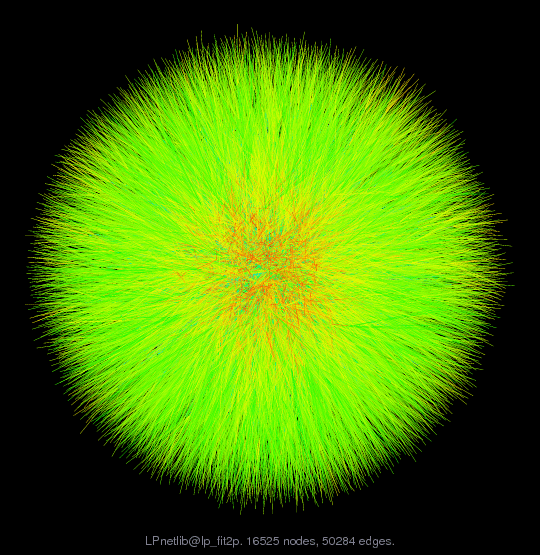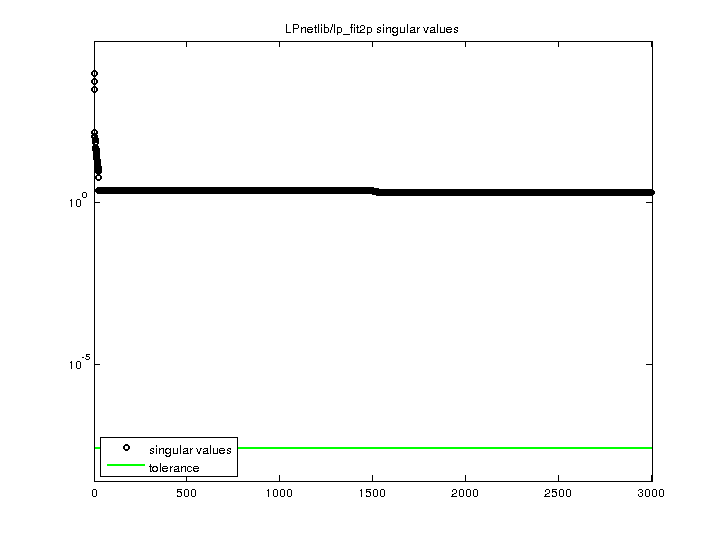Matrix: LPnetlib/lp_fit2p

Description: Netlib LP problem fit2p: minimize c'*x, where Ax=b, lo<=x<=hi(bipartite graph drawing)• Matrix group: LPnetlib
• download as a MATLAB mat-file, file size: 102 KB. Use UFget(627) or UFget('LPnetlib/lp_fit2p') in MATLAB.

 Matrix properties number of rows 3,000 number of columns 13,525 nonzeros 50,284 structural full rank? yes structural rank 3,000 # of blocks from dmperm 1 # strongly connected comp. 1 explicit zero entries 0 nonzero pattern symmetry 0% numeric value symmetry 0% type real structure rectangular Cholesky candidate? no positive definite? no

 author R. Fourer editor R. Fourer date 1990 kind linear programming problem 2D/3D problem? no

 Additional fields size and type b full 3000-by-1 c full 13525-by-1 lo full 13525-by-1 hi full 13525-by-1 z0 full 1-by-1

Notes:

```A Netlib LP problem, in lp/data.  For more information
send email to netlib@ornl.gov with the message:

send index from lp

The following are relevant excerpts from lp/data/readme (by David M. Gay):

The column and nonzero counts in the PROBLEM SUMMARY TABLE below exclude
slack and surplus columns and the right-hand side vector, but include
the cost row.  We have omitted other free rows and all but the first
right-hand side vector, as noted below.  The byte count is for the
MPS compressed file; it includes a newline character at the end of each
line.  These files start with a blank initial line intended to prevent
mail programs from discarding any of the data.  The BR column indicates
whether a problem has bounds or ranges:  B stands for "has bounds", R
for "has ranges".  The BOUND-TYPE TABLE below shows the bound types
present in those problems that have bounds.

The optimal value is from MINOS version 5.3 (of Sept. 1988)
running on a VAX with default options.

PROBLEM SUMMARY TABLE

Name       Rows   Cols   Nonzeros    Bytes  BR      Optimal Value
FIT2P      3001  13525    60784     439794  B     6.8464293232E+04

BOUND-TYPE TABLE
FIT2P      UP

Supplied by Bob Fourer.

Concerning FIT1D, FIT1P, FIT2D, FIT2P, Bob Fourer says
The pairs FIT1P/FIT1D and FIT2P/FIT2D are primal and
dual versions of the same two problems [except that we
have negated the cost coefficients of the dual problems
so all are minimization problems].  They originate from
a model for fitting linear inequalities to data, by
minimization of a sum of piecewise-linear penalties.
The FIT1 problems are based on 627 data points and 2-3
pieces per primal pl penalty term.  The FIT2 problems
are based on 3000 data points (from a different sample
altogether) and 4-5 pieces per pl term.

Added to Netlib on  31 Jan. 1990

```

 Ordering statistics: result nnz(V) for QR, upper bound nnz(L) for LU, with COLAMD 14,846,550 nnz(R) for QR, upper bound nnz(U) for LU, with COLAMD 4,501,500

 SVD-based statistics: norm(A) 9377.31 min(svd(A)) 2 cond(A) 4688.65 rank(A) 3,000 sprank(A)-rank(A) 0 null space dimension 0 full numerical rank? yes

 singular values (MAT file): click here SVD method used: s = svd (full (A)) ; status: ok